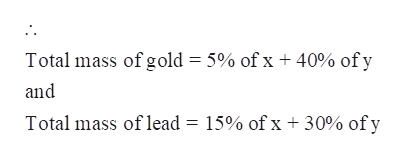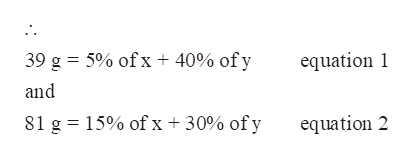# A chemist has two alloys, one of which is 5% gold and 15% lead and the other which is 40% gold and 30% lead. How many grams of each of the two alloys should be used to make an alloy that contains 39 g of gold and 81 g of lead?first alloy    gsecond alloy    g

Question
3 views

A chemist has two alloys, one of which is 5% gold and 15% lead and the other which is 40% gold and 30% lead. How many grams of each of the two alloys should be used to make an alloy that contains 39 g of gold and 81 g of lead?

 first alloy g second alloy g
check_circle

Step 1

A chemist has two alloys, one of which is 5% gold and 15% lead and the other which is 40% gold and 30% lead. The grams of each of the two alloys that should be used to make an alloy that contains 39 g of gold and 81 g of lead are to be calculated.

Step 2

Let x be the total amount of Alloy 1 and y be the total amount of alloy 2 that should be used to make an alloy that contains 39 g of gold and 81 g of lead .

Now, Alloy 1 has 5% gold and 15% lead.

And alloy 2 has 40% gold and 30% lead.help_outlineImage TranscriptioncloseTotal mass of gold 5% of x + 40% of y and Total mass of lead = 15% of x + 30% of y fullscreen
Step 3

Now,

The mass of gold in new alloy = 39 g

And the...help_outlineImage Transcriptionclose39 g 5% of x + 40% of y equation and 81 g 15% of x 30% of y equation 2 fullscreen

### Want to see the full answer?

See Solution

#### Want to see this answer and more?

Solutions are written by subject experts who are available 24/7. Questions are typically answered within 1 hour.*

See Solution
*Response times may vary by subject and question.
Tagged in

### Chemistry